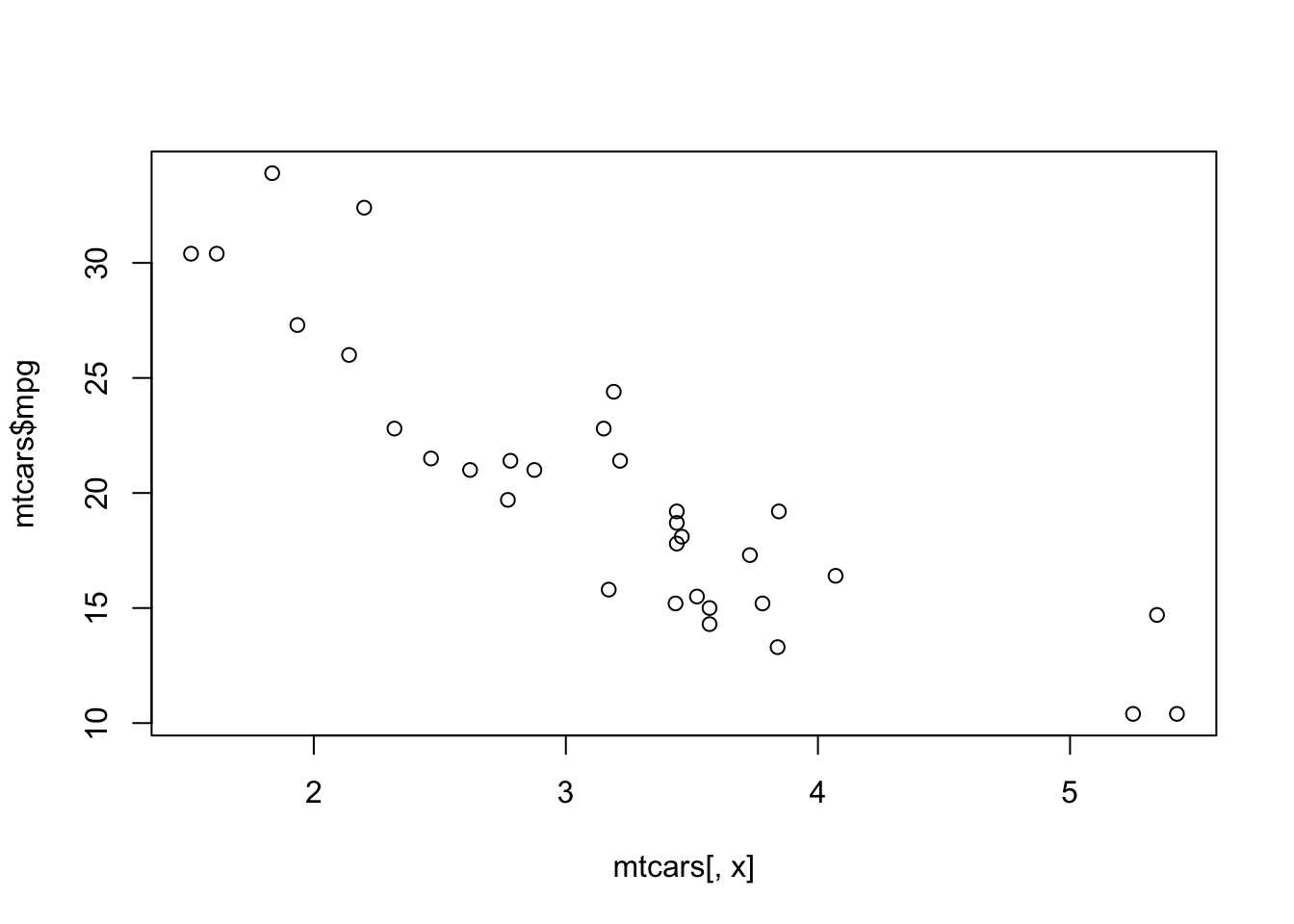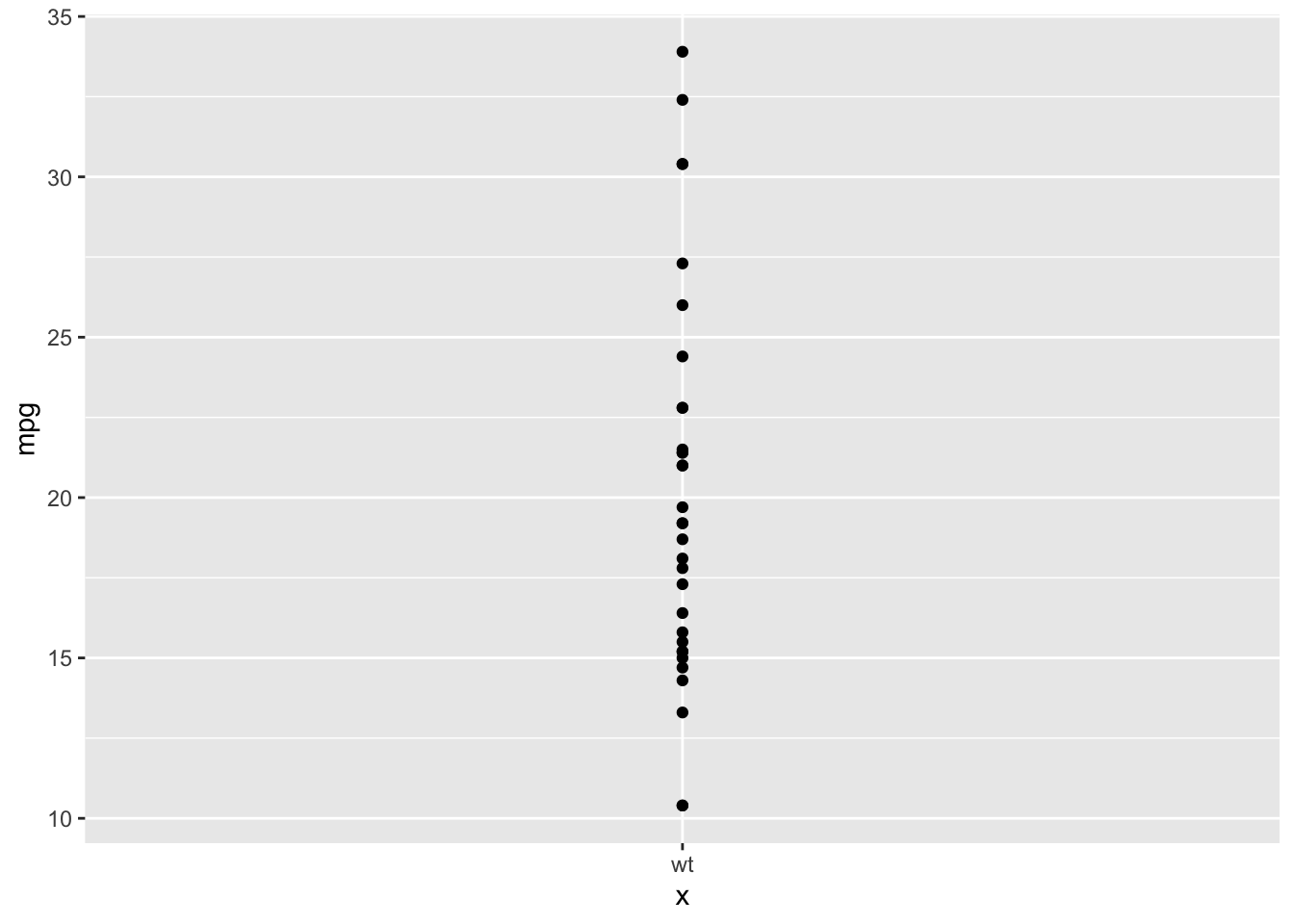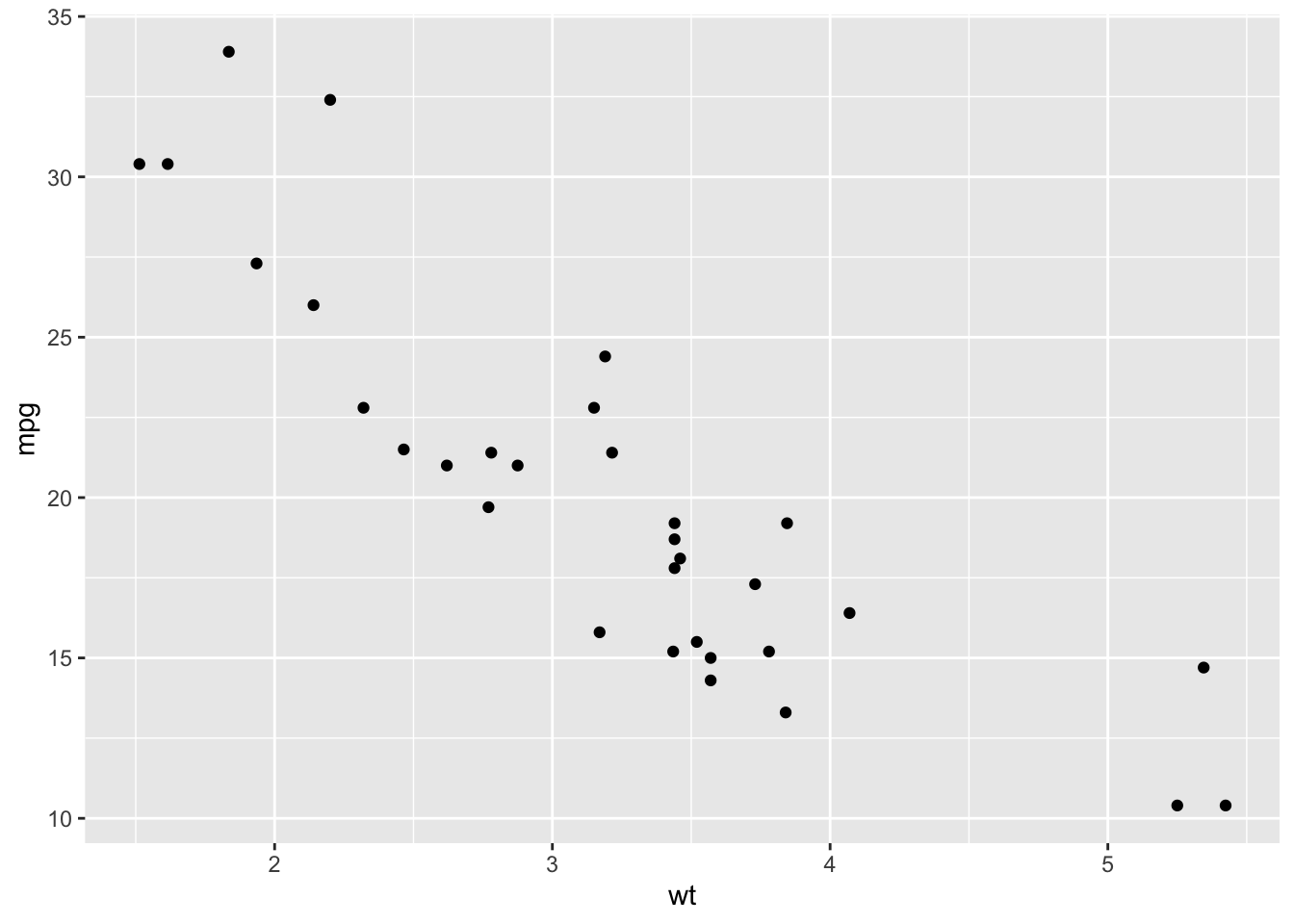# 4 Outputs

Once we have user input, it is time to tell our app what to do with these values. One way of doing this is calling `input` objects within a `render*()` function.

## 4.1 render*() functions

Objects from `input` (created in `ui`) can be called and operated on within a `render*()` function and stored in `output` (in `server`), and then called with an `*Output()` function (in `ui`).

Each `*Output()` function is paired with a `render*()` function:

`render*()` function `*Output()` function Uses
`renderTable()` `tableOutput()` Simple tables
`renderDataTable()` `dataTableOutput()` Feature-rich tables with search, filter, and pagination
`renderImage()` `imageOutput()` Images
`renderPlot()` `plotOutput()` Plots
`renderPrint()` `verbatimTextOutput()` Text in the style of that returned by `print()`
`renderText()` `textOutput()` “Normal” text
`renderUI()` `uiOutput()`, `htmlOutput()` Dynamic outputs, HTML code

Examples of the first six pairs of `render*()` and `*Output()` functions can be seen in the app below. To see the source code, click here to view the full app.

## 4.2 Example App

The app produced by the code below allows users to filter the `mtcars` data by values of `cyl`, and then it plots the raw data and fits a simple regression. In practice, fitting models is not a good idea for Shiny apps. Even fairly basic models with small datasets can take minutes to fit, which is a long time for a user to wait for a webpage. Instead, try returning summary statistics such as means or ranges, or if you are set on returning regression results, have a few models pre-fit and saved as data files, and then read these in and display them in the app.

Copy the code below into a script and save it to run the app. Observe how `renderPlot()` is paired with `plotOutput()`, and `renderText()` with `textOutput()`.

``````library(shiny)
library(dplyr)
library(ggplot2)

ui <-
fluidPage(
checkboxGroupInput("cyls",
"Number of cylinders:",
choices = c(4, 6, 8),
selected = c(4, 6, 8)),

plotOutput(outputId = "myPlot"),

textOutput(outputId = "myText")
)

server <-
function(input, output) {

output\$myPlot <-
renderPlot({
dat <- mtcars |> filter(cyl %in% input\$cyls)
coefs <- lm(mpg ~ wt, data = dat) |> coef()

ggplot(dat, aes(wt, mpg)) +
geom_point() +
geom_abline(intercept = coefs, slope = coefs, linetype = 2)
})

output\$myText <-
renderText({
dat <- mtcars |> filter(cyl %in% input\$cyls)
coefs <- lm(mpg ~ wt, data = dat) |> coef()

paste("The line has intercept",
round(coefs, 2),
"and slope",
round(coefs, 2)
)
})

}

shinyApp(ui = ui, server = server, options = list(display.mode = "showcase"))``````

Note that instead of providing coefficients to `geom_abline()`, we could have had ggplot calculate the best fitting line for us with `geom_smooth(method = "lm", se = F)`.

Also note that the `render*()` functions also have curly braces (`{}`) inside. They are only required if more than one expression is inside, but it is best practice to always include them. Here, both `renderPlot()` and `renderText()` each have three expressions (`dat <- ...`, `coefs <- ...`, and `ggplot(...` or `paste(...`), so they are required.

Each `render*()` function stores its result in `output\$outputId` in `server`, and this is accessed by `ui` as just `outputId` within an `*Output()` function, such as how `plotOutput()` accesses `output\$myPlot` with `plotOutput("myPlot")`.

While this app works fine enough to produce a plot and return the intercept and slope of a simple linear regression, you may notice some problems with the app:

• How many times did we filter the same data and fit the same model? (We will learn how to fix this in Reactivity.)
• What happens if you uncheck all the options in the input? (We will revisit this in Error Messages.)

## 4.3 Responsive Inputs

The functions in the final line of the table above, `renderUI()` plus `uiOutput()` or `htmlOutput()`, can be used to create reactive outputs. `uiOutput()` is just an alias for `htmlOutput()`, so the two functions can be used interchangeably. One of their uses is to create inputs whose values change in response to another input.

You have probably encountered such inputs when filling out your address in an online form. A first question may have asked you to pick your state of residence from a menu, and then the second question asked you to pick your county. Depending on which state was selected, different values of county appeared in the second question.

Two examples of responsive inputs can be viewed below or by clicking here.

Let’s look at the parts of the app that were used to create the first input pair.

``````library(shiny)

ui <-
fluidPage (
label = "Pick a region of the US",
choices = levels(state.region)),

uiOutput(outputId = "myUI")
)

server <-
function(input, output) {
output\$myUI <-
renderUI({
selectInput(inputId = "state",
label = "Pick a state in that region:",
choices = state.name[state.region == input\$region])
})
}

shinyApp(ui = ui, server = server, options = list(display.mode = "showcase"))``````

In this code, the value of `input\$region` from `radioButtons()` in `ui` is passed to `selectInput()` within `renderUI()` in `server`. The input control is then returned to `uiOutput()` in `ui`. In contrast to the outputs we have previously seen, responsive outputs can be interacted with and pass their values onto other `render*()` functions.

The next step, then, would be to use the value of `input\$state` in a `render*()` function, which would return an object to the output.

## 4.4 Character Data

Many `*Input()` functions return character vectors, and these can be tricky to use. In the app we created, `selectInput()` returns a character vector, and our plot call is `plot(mtcars[, input\$xvar], mtcars\$mpg)`. Different extractors (`[]`, `\$`) require different data types.

### 4.4.1 Variables

For example, we can create a simple character vector with length one called `x`, and make its value `"wt"`, one of the column names in `mtcars`. First note that we cannot extract `mtcars\$wt` with `mtcars\$x`. We must use the square brackets (`[]`). On the other hand, tidyselect functions like `pull()` or `select()` accept character vectors, so no special notation is needed.

``````library(dplyr)

x <- "wt"

mtcars\$x``````
``## NULL``
``mtcars[, x]``
``````##   2.620 2.875 2.320 3.215 3.440 3.460 3.570 3.190 3.150 3.440 3.440 4.070
##  3.730 3.780 5.250 5.424 5.345 2.200 1.615 1.835 2.465 3.520 3.435 3.840
##  3.845 1.935 2.140 1.513 3.170 2.770 3.570 2.780``````
``mtcars |> pull(x)``
``````##   2.620 2.875 2.320 3.215 3.440 3.460 3.570 3.190 3.150 3.440 3.440 4.070
##  3.730 3.780 5.250 5.424 5.345 2.200 1.615 1.835 2.465 3.520 3.435 3.840
##  3.845 1.935 2.140 1.513 3.170 2.770 3.570 2.780``````

As we have already seen, to plot in base R, we should use square brackets rather than the dollar sign. For ggplot, the notation is a bit more complicated. ggplot requires variable names as aesthetics. We have to first convert the character string to a symbol with `sym()` and then force it to be evaluated as such with the bang-bang operator (`!!`): `!!sym(x)`.

``````x <- "wt"
plot(mtcars\$x, mtcars\$mpg) # incorrect``````
``## Error in xy.coords(x, y, xlabel, ylabel, log): 'x' and 'y' lengths differ``
``plot(mtcars[, x], mtcars\$mpg) # correct````````library(ggplot2)
ggplot(mtcars, aes(x, mpg)) + geom_point() # incorrect````````ggplot(mtcars, aes(!!sym(x), mpg)) + geom_point() # correct``### 4.4.2 Formulas

`lm()` and other modeling functions also have special requirements that the formula argument must be of class formula, not of class character. To change the data type to formula, simply pass a character string to `as.formula()`.

``````x <- "wt"
myFormula <- as.formula(paste("mpg ~", x))
myFormula``````
``## mpg ~ wt``
``lm(myFormula, data = mtcars)``
``````##
## Call:
## lm(formula = myFormula, data = mtcars)
##
## Coefficients:
## (Intercept)           wt
##      37.285       -5.344``````

If one or more variables are to be supplied to a formula, as might be the case with `checkboxGroupInput()` (e.g., “Check the predictors to include in the model”), the `collapse` argument of `paste()` comes in handy. We can use `collapse = "+"` to take all the elements from `x` and combine them into a single element, inserting `+` between each one.

``````x <- c("wt", "hp", "disp")
myFormula <- as.formula(paste("mpg ~",
paste(x, collapse = "+")
)
)
myFormula``````
``## mpg ~ wt + hp + disp``
``lm(myFormula, data = mtcars)``
``````##
## Call:
## lm(formula = myFormula, data = mtcars)
##
## Coefficients:
## (Intercept)           wt           hp         disp
##   37.105505    -3.800891    -0.031157    -0.000937``````

To see which data type a specific `*Input()` function returns, check the “Server value” section in the documentation.

## 4.5 Exercises

Copy and paste the following code into a new R script and save it. Click “Run App”. This app returns a plot of weight by MPG in the `mtcars` dataset, with weight filtered to be within a range picked by the user. Modify the app in these ways:

1. Add a table output of the filtered dataset.

2. Add a text output with summary statistics of `mpg` after filtering, such as its mean (`mean()`), standard deviation (`sd()`), or interquartile range (`IQR()`).

``````library(shiny)
library(dplyr)
library(ggplot2)

ui <-
fluidPage(
sliderInput(inputId = "range",
label = "Pick a range for mtcars\$wt",
min = 1.5,
max = 5.5,
value = c(1.5, 5.5)),

plotOutput(outputId = "myPlot")
)

server <-
function(input, output) {
output\$myPlot <-
renderPlot({
dat <-
mtcars |>
filter(wt >= input\$range,
wt <= input\$range)

ggplot(dat, aes(wt, mpg)) +
geom_point()
})

}

shinyApp(ui = ui, server = server)``````

Challenge:

Follow up on the idea in the end of the section on Responsive Inputs. Use the value of `input\$state` in another output. Copy the code below into an R script and save it to create an app. Add a `renderText()` in `server` that returns a fact about the selected state (`input\$state`) from the `state.x77` dataset, such as its population, which you can retrieve with `state.x77[input\$state, "Population"]`. Then, display this text with a `textOutput()` in `ui`.

``````library(shiny)

ui <-
fluidPage (
"Pick a region of the US",
levels(state.region)),

uiOutput(outputId = "myUI")
)

server <-
function(input, output) {
output\$myUI <-
renderUI({
selectInput(inputId = "state",
label = "Pick a state in that region:",
choices = state.name[state.region == input\$region])
})
}

shinyApp(ui = ui, server = server, options = list(display.mode = "showcase"))``````

1. Use the values from the inputs created in the previous exercises in at least two `render*()` functions.
2. Create an output for each `render*()` function.